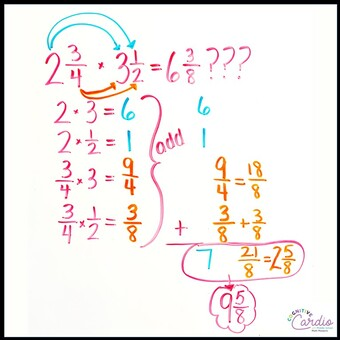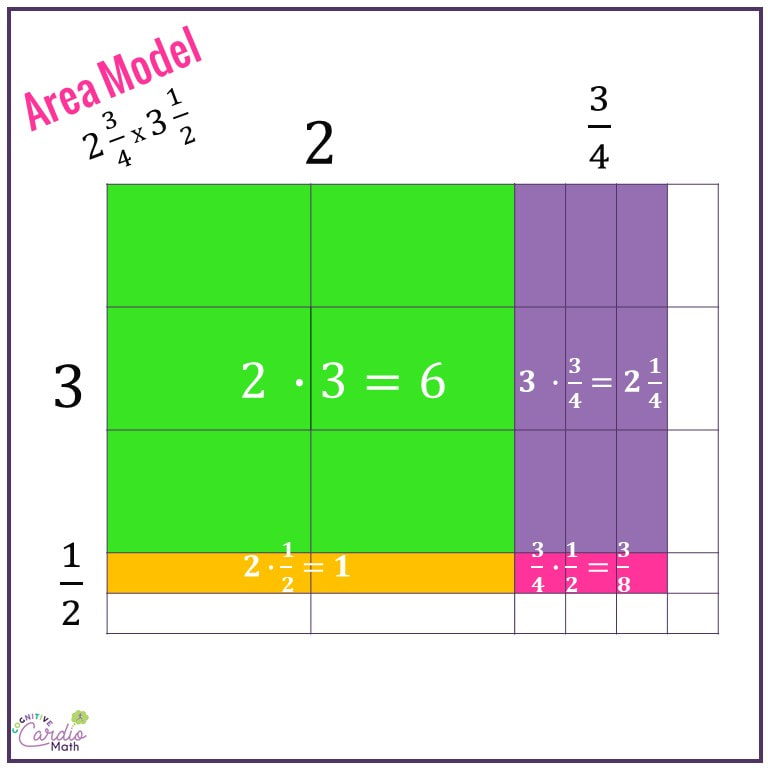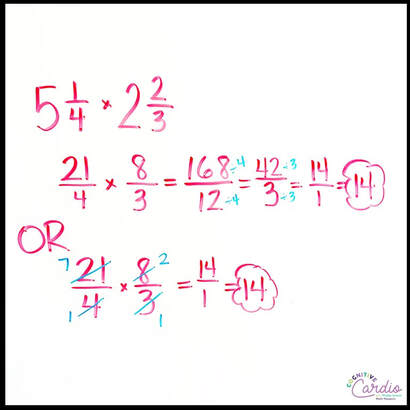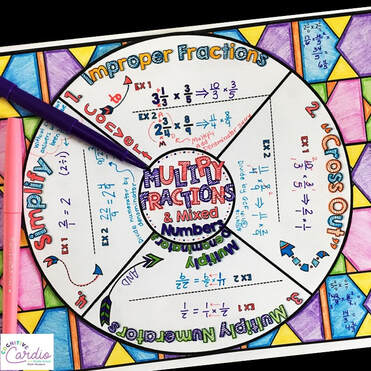# Teaching Multiplication of Fractions and Mixed Numbers

Three Tips for Teaching Fraction MultiplicationHow much do your 6th grade math students love multiplying mixed numbers?? If they’re like mine, they might not love it at first:-)

I love teaching fraction multiplication in middle school math–and I especially love teaching multiplication of mixed numbers. Why?
Because we have fun exploring why multiplying mixed numbers DOESN’T work a certain way.

## Multiplying Mixed Numbers

Inevitably, when we start multiplying mixed numbers (which students have done in 5th grade math, but we review in 6th grade), some students want to multiply the fractions by the fractions and then multiply the whole numbers by the whole numbers.

And I can see why they might think that’s ok – after all, when they add and subtract fractions, they add/subtract the whole numbers and fractions separately.

## Multiplying Mixed Numbers Tip #1:Show What Doesn’t Work and WHYSo, every year, we end up having a discussion about why the method of multiplying fractions by fractions and whole numbers by the whole numbers just doesn’t work.

We discuss how multiplying 2_3/4 by 3_1/2 means that ALL parts of 2_3/4 must be multiplied by ALL parts of 3_1/2. On the board, we make a list of the problems that would need to be completed to make sure all parts are multiplied:

• 2 x 3
• 2 x 1/2
• 3/4 x 3
• 3/4 x 1/2.• Once we complete those problems, students can see 6 and 3/8 in that list of products, which would be the answer if they multiplied fraction by fraction and whole number by whole number.
•
• However, they can now see that there’s also an additional 1 and 9/4 in the list, which are part of the final answer.Once we look at all four products, we go through the process of adding them all together (finding common denominators, equivalent fractions, etc) and then simplifying…..quite a bit of work to get to the answer:-)It may also help to construct a visual model for students, especially if they still don’t have a great grasp of what these smaller multiplication problems mean.

Seeing the visual model and the calculations side by side will make it more concrete for them.

Another visual for students to use is the area model.

The area model puts all the parts from the visual above into one model, which shows the whole numbers and fractional parts.

Students can see the representation of the 4 problems that make up the entire product (below).## Convert Mixed Numbers to Improper FractionsAfter we break down the problem to understand what it means, we compare that process and answer to what we get when we convert the mixed numbers to improper fractions (fractions greater than one).

We discuss the idea that when you convert a mixed number to an improper fraction, the new fraction now includes all the parts of the mixed number (so 11/4 includes the 2 and the 3/4).

The detailed example of completing four multiplication problems and adding the products proves not only that converting to improper fractions is necessary, but also that it’s a WHOLE lot faster!

So, Tip #1 is to show students WHY multiplying fraction by fraction and whole number by whole number doesn’t work. Break up the problem and demonstrate what the multiplication really means.

## Multiplying Fractions and Mixed Numbers Tip #2:Teaching Cross Canceling, or "Crossing Out"

Teach students to cross cancel, or “cross out”  (or whatever you might call it), and show them why it makes the fraction multiplication process a little easier.In recent years, I’ve found that students aren’t learning this in earlier grades as often as they used to–for many, the discussion we have in my classroom is the first time they’ve encountered it.

I love teaching this aspect of fraction multiplication. It’s hard for some students to grasp at first, but when they repeatedly see that if they don’t cross out, they have more simplifying at the end of the problem (with larger numbers, like 168/12), they start getting excited about finding how much they can cross out.

Once I teach them the idea of simplifying through crossing out, and we explore why it works, there are some that still want to stick with what they learned in earlier grades and simplify only at the end, while others get super-excited about the concept of making the numbers they’re working with smaller at the start.

I may be wrong on this, but it seems that the students who embrace it first are those who know their multiplication facts better and can more easily find the relationships between the numbers in the problem.
For example, a student who knows that 15 and 24 are both divisible by 3 is more likely to go ahead ‘cross out’ by dividing them by 3 than the student who can’t see it because they can’t remember or don’t know what 15 and 24 are divisible by.

## Multiplying Fractions and Mixed Numbers Tip #3:Use a Graphic Organizer

While the process of multiplying fractions can be easy to remember, it can be difficult for some students to remember the process of multiplying mixed numbers.

Giving students a graphic organizer to help them remember the process can really help.Some will need this and some won’t, but it’s handy for them to keep in their binders to reference throughout the year.

I recently created a fun multiplication of fractions and  mixed numbers math wheel, which is a great way to have students take notes about the concept, practice it, and then add their own personal, artistic touches.

Do you have any special methods you use to teach the multiplication of fractions and mixed numbers?

## Fraction Operations Notes

Do your students need a little help in remembering the processes for all the operations. They can use this fraction operations wheel to keep the operations ‘straight.’You can grab this fraction wheel for free (plus a bunch of other fraction goodies) when you join my email community!

Interested in more fraction and fraction operation content?
Check out the program,

## EllieWelcome to Cognitive Cardio Math! I’m Ellie, a wife, mom, grandma, and dog ‘mom,’ and I’ve spent just about my whole life in school! With nearly 30 years in education, I’ve taught:

• All subject areas in 4th and 5th grades
• Math, ELA, and science in 6th grade (middle school)

I’ve been creating resources for teachers since 2012 and have worked in the elearning industry for about five years as well!